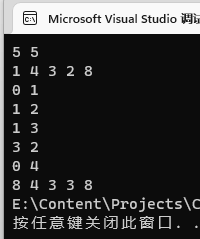superlevin 2022-05-12 20:58 采纳率: 100%浏览 57

# DAG演算法透過C++實踐

Here is a Directed Acyclic Graph (DAG) with weight on nodes.

If there is a directed path from node to node , node is a successor of node . itself is deﬁned as one of the successors of as well.
Please calculate the maximal weight among weights of all successors of for each in the DAG.

The ﬁrst line is n,m , which are number of nodes and directed edges in the DAG.
The following n line are n integers representing weight of node , i0≤i<.
The following m line after weights are m pair of integers(a,b) , which represents directed edge from a to b .
Constraints
0<n<=10000
0<m<=50000
0<wieght<=10000
0<=a,b<n
Output Format
Print n integers in a line separate with a space.

The i-th integer is the answer corresponding to node i 0<=i<n
Sample Input
4 4
1 4 3 2
0 1
1 2
1 3
3 2
Sample Output
4 4 3 3
Sample Input
5 5
1 4 3 2 8
0 1
1 2
1 3
3 2
0 4
Sample Output
8 4 3 3 8

``````
#include <cstdio>
#include <vector>
#include <iostream>
#include <algorithm>
using namespace std;
int main() {
/* Enter your code here. Read input from STDIN. Print output to STDOUT */
return 0;
｝
``````
• 写回答

#### 1条回答默认 最新

•丨秋水丨 2022-05-12 21:54
关注``````#include <cstdio>
#include <vector>
#include <iostream>
#include <algorithm>
using namespace std;
struct Node
{
int weight;
std::vector<int> successors;
};
int main() {
int n, m;
cin >> n >> m;
std::vector<Node> nodes;
nodes.resize(n);
for (int i = 0; i < n; ++i)
{
int w;
cin >> w;
nodes[i].weight = w;
}
for (int i = 0; i < m; ++i)
{
int l, r;
cin >> l >> r;
nodes[l].successors.push_back(r);
}
for (int i = 0; i < n; ++i)
{
int maxWeight = nodes[i].weight;
for (int j = 0; j < nodes[i].successors.size(); ++j)
{
if (maxWeight < nodes[nodes[i].successors[j]].weight)
{
maxWeight = nodes[nodes[i].successors[j]].weight;
}
}
cout << maxWeight;
if (i != n - 1)cout << " ";
}
return 0;
}
``````
本回答被题主选为最佳回答 , 对您是否有帮助呢?
评论 编辑记录

#### 悬赏问题

• ¥15 Pycharm无法自动补全，识别第三方库函数接收的参数！
• ¥15 STM32U575 pwm和DMA输出的波形少一段
• ¥30 android百度地图SDK海量点显示标题
• ¥15 windows导入environment.yml运行conda env create -f environment_win.yml命令报错
• ¥15 这段代码可以正常运行，打包后无法执行，在执行for内容之前一直不断弹窗，请修改调整
• ¥15 C语言判断有向图是否存在环路
• ¥15 请问4.11到4.18以及4.27和4.29公式的具体推导过程是怎样的呢
• ¥20 将resnet50中的卷积替换微ODConv动态卷积
• ¥15 通过文本框输入商品信息点击按钮将商品信息列举出来点击加入购物车商品信息添加到表单中
• ¥100 这是什么压缩算法？如何解压？